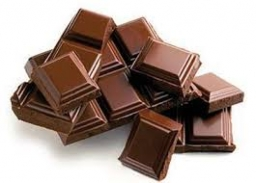# Chocolates 47241

Two chocolates and five teas cost a total of 498 CZK. Chocolate is 18 CZK more expensive than tea. How much do one chocolate and one tea cost?

a =  84
b =  66

### Step-by-step explanation:

2a+5b=498
a=18+b

2·a+5·b=498
a=18+b

2a+5b = 498
a-b = 18

Row 2 - 1/2 · Row 1 → Row 2
2a+5b = 498
-3.5b = -231

b = -231/-3.5 = 66
a = 498-5b/2 = 498-5 · 66/2 = 84

a = 84
b = 66

Our linear equations calculator calculates it.Did you find an error or inaccuracy? Feel free to write us. Thank you!

Tips for related online calculators
Do you have a system of equations and looking for calculator system of linear equations?